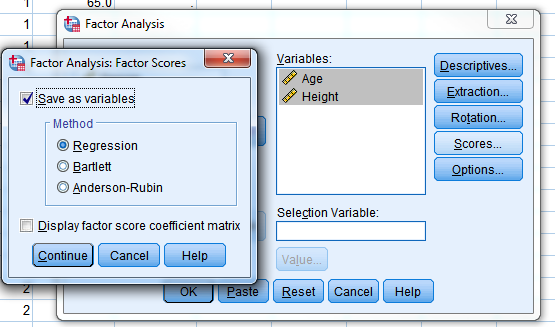Howdy, Stranger!

It looks like you're new here. If you want to get involved, click one of these buttons!

Supported by

Factor extraction

Hi folks
For example, when running ANCOVA it is sometimes useful to extract a single factor from 33 or so variables and then use this as the covariate. I have looked around and cannot see such an option currently in JASP. Am I missing something or is there an r code that could be used in the compute columns options?

Cheers
Mark

• Hi Mark,

When you say "extract a single factor", do you refer to factor analysis or just to averaging?

Cheers,
E.J.

• Hi EJ - looking at data reduction in effect.
Eg comparing brain size in males and females, combining data such as height, body mass etc into one column of data to use as a covariate factor

• I recall discussing this before, at least when the combination rule is of a simple form (e.g., a weighted average over the columns). Assuming that this is what you mean, I'll ask some team members for advice.

• This is the method in SPSS• Ah, so we need to be able to save the factor scores as a separate column. I think that this should in principle be doable; if you GitHub this then I can ask those responsible for the factor analysis code to do this (maybe it is already done, at least in the developer's version).
Cheers,
E.J.

• Looks like this was raised in Nov 2016 on GitHub but nothing more since.
Cheers
Mark

• We've made a lot of progress since then, so I think we are in a good position to address this issue now.

• If I understand the question correctly than with the new version and a computed column this should be possible.

What I understand, taking the shown example, is that two variables (Age & Height) must be combined in some way to create a single variable. This could be done through some arithmetic function as addition or something. Or one could want to have them categorized into a nominal perhaps?

Assuming the arithmetic option one can create an "Scalar R Computed Column" and then use (a variation of) the following R code:
Age + Height or Age * Height or something.

This will obviously become a bit more unwieldy with more variables and is thus perhaps not optimal. But certainly functional. And to get this normalized to some constraint you could then take a second computed column that uses your first as an input.

We are thinking of making it easier to select groups of columns and combining them in the computed columns and filter. But are unsure yet how to proceed. Any feedback is welcome.

• This video explains it I believe - from 2.10min onwards

Cheers
Mark